# Osmotic Pressure Formula

Osmotic Pressure Formula

If two solutions of different concentrations are separated by a semipermeable membrane (a membrane that only allows water to pass) osmosis will occur. The solution with a lower concentration will pass water through the membrane to the solution with the higher concentration.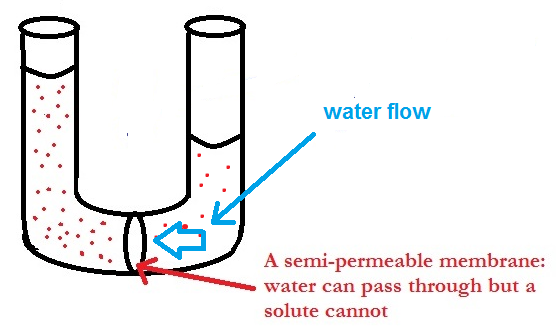The process will continue until either the concentrations of the two solutions are the same or if the added pressure (caused by the extra water) becomes too great for any more water to pass through the membrane. This added pressure is known as the osmotic pressure or the pressure it takes to stop osmosis.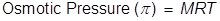M = the molar concentration of the solution

R = the gas laws constant (0.0821)

T = the Kelvin temperature

Osmotic Pressure Formula Questions:

1. What is the osmotic pressure of a 2.75 Molar glucose solution at 25°C?

In order to change the Celsius temperature to Kelvin, 273 must be added to Celsius value.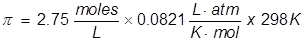2. What is the molarity of a sucrose solution if the osmotic pressure is 1.35 atm at 35°C?

In order to calculate the Molarity, the temperature needs to be changed to Kelvin and the equation will be rearranged.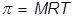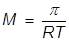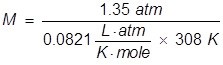Related Links: Gases: Pressure Quiz Carl Bosch Facts Density Examples Pneumatics Examples Pressure Formula Gay-Lussac's Law Formula Boyle's Law Formula Ideal Gas Law Formula